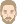## The difference between cloud index and Okta

WEI LI
WUFI UserPosts: 35
Joined: Thu Oct 29, 2020 1:02 pm -1100

### The difference between cloud index and Okta

Hi experts,

The cloud data from the weather station here in New Zealand is in the formate of Okta, which is in the range of 0-8, with 0 representing the sky is clear and 8 representing the sky is fully covered.
To switch the Okta data to the cloud index in WUFI, all I have to do is switch the scale from eight to one. 8 in Okta represents 1 in the cloud index.
Is that correct?
Thank you!

WEI LI
WUFI UserPosts: 35
Joined: Thu Oct 29, 2020 1:02 pm -1100

### Re: The difference between cloud index and Okta

Besides, data in the weather station shows that at a specific hour, it may have several layers of cloud, each layer has its own oktas value.
Cheers

ThomasPosts: 365
Joined: Sun Jun 19, 2005 10:33 pm -1100

### Re: The difference between cloud index and Okta

WEI LI wrote:
Thu Apr 21, 2022 6:43 pm -1100
To switch the Okta data to the cloud index in WUFI, all I have to do is switch the scale from eight to one. 8 in Okta represents 1 in the cloud index.
Is that correct?
Yes, the cloud index in WUFI is the fraction of the sky which is covered with clouds, so simply divide the Okta's by eight.

(Be careful to check for the possible occurrence of values like 9 Okta. Depending on the conventions of the weather station they may mean something like "not observed" and must not be converted to a cloud cover 1.125).
Besides, data in the weather station shows that at a specific hour, it may have several layers of cloud, each layer has its own oktas value.
WUFI uses the cloud cover to estimate the long-wave atmospheric counterradiation if the simulation is to be done with activated "explicit radiation balance" (for which counterradiation data are needed) and if no measured counterradiation is available. No cloud cover data are needed if the simulation is run in standard mode, or if measured counterradiation data can be used.

WUFI uses a very simple model to estimate the long-wave atmospheric counterradation from cloud cover data. The counterradiation is the thermal radiation emitted by the sky. The atmospheric gases are poor thermal emitters, so the contribution of patches of clear sky is small. Thick, low clouds are good emitters, so they contribute a larger amount of radiation (if they exist).

WUFI uses the following model for clear sky [Lit. 1]:

$$A_\mathrm{k} = \sigma \ T_\mathrm{2 m}^4 \ (0.790 - 0.174 \cdot 10^{-0.041 \ e})$$

$$A_\mathrm{k}$$: atmospheric counterradiation from clear sky, $$\mathrm{W/m^2}$$
$$\sigma$$: Stefan-Boltzmann constant, $$5.670 \cdot 10^{-8} \ \mathrm{W/(m^2K^4)}$$
$$T_\mathrm{2 m}$$: absolute station temperature at 2 meters, $$\mathrm{K}$$
$$e$$: water vapour partial pressure at the station, $$\mathrm{hPa} (!)$$

WUFI uses the following model for cloudy sky [Lit. 1]:

$$A_\mathrm{w} = \sigma \ T_\mathrm{dew, 2 m}^4$$

$$A_\mathrm{w}$$: atmospheric counterradiation from cloudy sky, $$\mathrm{W/m^2}$$
$$T_\mathrm{dew, 2 m}$$: absolute dew point temperature at 2 meters, $$K$$

This model assumes that the bottom of the clouds has dew point temperature. This is a good assumption for low, thick clouds created by convection. It's not so good for higher, cooler clouds.

The total counterradation is the sum of the contributions from clear and cloudy patches weighted by the cloud cover fraction:

$$A = N \cdot A_\mathrm{w} + (1-N) \cdot A_\mathrm{k}$$

$$N$$: cloud cover (fraction of the sky covered with clouds), $$-$$

.

There are also models which take the different contributions of clouds at different heights into account. For example, there is the model derived by Czeplak and Kasten [Lit. 2]. They start with the Swinbank model for a clear sky

$$A_\mathrm{k} = \varepsilon_\mathrm{eff} \ \sigma \ T_\mathrm{2 m}^4$$

$$A_\mathrm{k}$$: clear-sky atmospheric counterradiation, $$\mathrm{W/m^2}$$
$$\varepsilon_\mathrm{eff} = 9.9 \cdot 10^{-6} \cdot (T/\mathrm{K})^2$$: effective emissivity of the atmosphere
$$\sigma$$: Stefan-Boltzmann constant, $$5.670 \cdot 10^{-8} \ \mathrm{W/(m^2K^4)}$$
$$T_\mathrm{2 m}$$: absolute station temperature at 2 meters, $$\mathrm{K}$$

and add the contributions from the various cloud layers

$$A = A_\mathrm{k} \cdot (1 + a_\mathrm{l} \cdot n_\mathrm{l}^{2.5} + a_\mathrm{m} \cdot (1-n_\mathrm{l}) \cdot n_\mathrm{m}^{2.5} + a_\mathrm{h} \cdot (1-n_\mathrm{l})\cdot(1-n_\mathrm{m})\cdot n_\mathrm{h}^{2.5})$$

where $$n_\mathrm{l}$$, $$n_\mathrm{m}$$ and $$n_\mathrm{h}$$ are the cloud cover fractions for low, medium and high clouds, respectively. Czeplak and Kasten find the coefficients

$$a_\mathrm{l} = 0.243$$
$$a_\mathrm{m} = 0.196$$
$$a_\mathrm{h} = 0.091$$

These coefficients show that the contribution of high (and cool) clouds is noticeably less.

Newer research has shown a small temperature dependence [Lit. 3][Lit. 4]:

$$a_\mathrm{l} = 2.30 - 7.37\cdot10^{-3} \cdot T_\mathrm{2 m}/K$$
$$a_\mathrm{m} = 2.48 - 8.23\cdot10^{-3} \cdot T_\mathrm{2 m}/K$$
$$a_\mathrm{h} = 2.89 - 1.00\cdot10^{-2} \cdot T_\mathrm{2 m}/K$$

These models are not implemented in WUFI. If you only have the layer-specific data, then as the simplest solution you could maybe try to combine the low and medium cloud fractions (these apparently being of comparable importance) in the above sense:

$$N \approx n_\mathrm{l} + (1-n_\mathrm{l}) \cdot n_\mathrm{m}$$

However, a more rigorous approach would be to use the layer-specific model to estimate the counterradiation, using all available data, and then - using the first model above - to compute for WUFI the single-layer cloud cover which produces the same counterradiation.

Kind regards,
Thomas

[Lit. 1]
Blümel K. et al.:
Entwicklung von Testreferenzjahren (TRY) für Klimaregionen der Bundesrepublik Deutschland
BMFT-Forschungsbericht T 86-051, 1986

[Lit. 2]
Czeplak G., Kasten F.:
Parametrisierung der atmosphärischen Wärmestrahlung bei bewölktem Himmel.
Meteorologische Rundschau, Heft 6 (1987), 184-187

[Lit. 3]
Christoffer J., Deutschländer Th., Webs M.:
Testreferenzjahre von Deutschland für mittlere und extreme Witterungsverhältnisse TRY
Selbstverlag DWD, Offenbach a. Main 2004

[Lit. 4]
VDI Guidelines
VDI 3789, Part 2: Environmental Meteorology: Interactions between Atmosphere and Surfaces - Calculation of Short-Wave and Long-Wave Radiation.
Beuth-Verlag